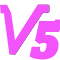计算轨道炮效率
设轨道的电感梯度为dL/dx，回路总电阻为R，储能为Es，弹丸质量为m，初速v0，设t1时弹丸速度为v，电流为I，平均效率为η。
其中，电阻R为回路中各个部分电阻之和。包括电源内阻，轨道电阻，开关电阻，接触电阻等。若有产生压降的部分，如等离子体电枢，则将压降折算为电阻。
易知电阻损耗功率
$$P_R=I^2R \hspace{1cm} (1)$$

$$I^2=\frac{P_R}{R} \hspace{1cm} (2)$$
电磁力
$$F=\frac{1}{2} I^2 \frac{dL}{dx} \hspace{1cm} (3)$$
若不考虑摩擦，则t1时弹丸速度满足
$$v=v_0+\frac{1}{m} \int_0^{t_1} F \, dt \hspace{1cm} (4)$$

$$v=v_0+\frac{1}{2m} \int_0^{t_1} I^2 \frac{dL}{dx} \, dt = v_0+\frac{1}{2m} \int_0^{t_1} \frac{P_R}{R} \frac{dL}{dx} \, \,dt \hspace{1cm} (5)$$
如果认为电阻R，和电感梯度dL/dx，在整个加速过程中均保持不变。（对于使用电解电容的方案，回路电阻主要集中在电容ESR上，故此等效误差不大）
则有
$$v= v_0+\frac{1}{2m} \int_0^{t_1} \frac{P_R}{R} \frac{dL}{dx} \, \,dt = v_0+\frac{E_R}{2mR} \, \frac{dL}{dx} \hspace{1cm} (6)$$
其中，$$E_R$$为消耗在回路电阻上的总能量。
弹丸动能
$$E_k = \frac{1}{2}mv^2 = \frac{ {E_R}^2 }{8mR^2}\,\left ( \frac{dL}{dx} \right)^2 + \frac{ {E_R} v_0}{2R}\,\frac{dL}{dx} + \frac{1}{2}m{v_0}^2 \hspace{1cm} (7)$$
弹丸动能的增量
$$\Delta E_k = E_k - E_{k0} =\frac{ {E_R}^2 }{8mR^2}\,\left ( \frac{dL}{dx} \right)^2 + \frac{ {E_R} v_0}{2R}\,\frac{dL}{dx}\hspace{1cm} (8)$$
其中，$$E_{k0}$$为弹丸的初始动能。
当$$E_k \ll E_R$$，即效率η趋近于0（比如η<10%）时，可以忽略弹丸动能$$E_k$$，因此有
$$\eta = \frac{\Delta E_k}{E_R+E_k} = \frac{\Delta E_k}{E_R} \hspace{1cm} (9)\\ \, \\E_R = E_S - E_k = E_s \hspace{1cm} (10)$$

$$\eta = \frac{ {E_S}}{8mR^2}\,\left ( \frac{dL}{dx} \right)^2 + \frac{ v_0}{2R}\,\frac{dL}{dx} \hspace{1cm} (11)$$
补充：式中各符号含义，Es为总储能，dL/dx为电感梯度，m为弹丸重量，R为回路总电阻，v0为弹丸初速。
重复一下这个式子的适用条件：忽略摩擦力，忽略回路电阻变化（包括等离子体“等效电阻”的变化），认为电感梯度为恒定值，不计轨道中剩余的磁能，效率趋近于零。
有趣的是，这里算出的效率与电流无关，无论是电流波形还是电流大小。当然，电流过大或过小时，摩擦，电阻变化等不可忽略，会导致这个式子不适用，这种情况下影响还是很大的。不过当电流在小范围内变化时，可以认为这个结论成立。

据此不难解释为何民间的轨道炮效率普遍不堪入目。
我们代入一组参数。轨道电感梯度为0.5uH/m^2，使用330V，10000uF电容储能，即能量约为540J，内阻约为3~5mΩ，以5mΩ计（忽略其他部分的电阻），使用重1g的弹丸，从静止开始加速，则根据上式，算得效率为0.0675%，已经可以以ppm计了……
但是如果把电容容量放大5倍，即50mF，那么此时电容储能约为2700J，内阻约为0.6~1mΩ，以1mΩ计（忽略其他部分电阻），其他条件相同的情况下，算得效率8.4%，相当可观。
也就是说高储能下高效率将会更容易达到，但是高储能刚好是多数业余爱好者不会去搞的……这是民间轨道炮效率普遍不佳的一个很重要的原因。当然，除了提高储能还有好多办法去提高效率，从上面的式子来看，只要提高分子，减小分母就好了……即提高电感梯度，减小回路电阻，减小弹丸重量，和提供初速（这几项爱好者通常做得也不太好），这几项的具体实现方法与本帖主题无关，这里就不多言了。

[修改于 3 年前 - 2016-10-04 16:09:36]

来自：高压与强磁 / 电磁炮

2
2016-9-6 1:26:34虎哥1楼
储能增加的同时往往伴随着电源内阻减小，所以影响相当明显。
初速如果大到某个程度，效率似乎可以超过100%，这到底是哪个因素导致公式不适用？即为什么要效率接近于零时才适用，能不能推出效率较高时的计算公式。
加载评论中，请稍候...三水合番（作者）
2楼
引用 虎哥:
储能增加的同时往往伴随着电源内阻减小，所以影响相当明显。
初速如果大到某个程度，效率似乎可以超过100%，这到底是哪个因素导致公式不适用？即为什么要效率接近于零时才适用，能不能推出效率较高时的计算公式……
的确有效率超100%的问题。因为顶楼的效率=动能/电阻损耗的能量。效率足够高的时候，动能可以超过电阻损耗，于是会效率超100%……
让效率=动能/（电阻损耗+动能），可以部分解决这个问题，但是会让公式显得很繁琐…而且效率高的时候，顶楼结论的部分前提条件（忽略电阻变化，忽略剩余磁能）无法满足，所以即使改成“效率=动能/（电阻损耗+动能）”，精度也会很差。所以干脆写成只适用于效率足够低时的简洁形式了。
如果能考虑到剩余磁能和电阻变化，就能推出效率较高时的计算公式，但是这两个东西用解析的方式不好算……数值分析倒是不错，不过那就不在这篇帖子的讨论范围了。
加载评论中，请稍候...
2016-09-10 22:20:10
2016-9-10 22:20:10三水合番（作者）
3楼
最近看到了一篇论文挺经典的一篇，截至目前，被引用次数63，在轨道炮的圈子里相当高了。
里面就对轨道炮的效率进行了计算，和顶楼不同，他用出速，电阻和电感梯度表示的效率。不过和顶楼相同的是，他也假设了回路电阻不变，对于需要考虑电阻变化的情况，他是这样一笔带过的：“An average system resistance can be used in these cases.”感觉也不太严谨，不过解析方法也就能做到这里了……至少用来算效率极限还是可以的。
我也来把顶楼的式子改一下，来凑凑热闹吧 ( ’◡’)

从顶楼式（8）开始，沿另一条路推下去。

$$E_k = \frac{1}{2}mv^2$$
可以得到
$$m = \frac{2E_k}{v^2}$$
设初速v0=0。将其代入顶楼式(7)，同时把弹丸质量用上面的式子进行替换。可以得到
$$\Delta E_k = E_k = \frac{v^2 {E_R}^2 L'^2}{16E_k R^2}$$
其中，L‘ = dL/dx，即电感梯度，表示还是这么写比较方便……
等式两边同时乘以Ek，然后同时开方，得到
$$E_k = \frac{v {E_R} L'}{4 R}$$
然后就是求效率，和顶楼不同，这里我们不再认为Ek可忽略，但是我们依旧忽略剩余磁能，以及摩擦力的影响。因此，可以得到效率
$$\eta = \frac{E_k}{E_k+E_R} = \frac{1}{1+\frac{E_R}{E_k}}$$

$$\eta = \frac{1}{1+\frac{4R}{vL'}}$$
式中，R为回路电阻，v为弹丸出速，L‘为轨道电感梯度。
适用条件为，回路电阻保持恒定，不考虑除回路电阻损耗以外的其他损耗。
和上面那篇论文里得到的结论相同。
那篇论文里，还定义
$$\sigma = \frac{4R}{L'}$$
$$\sigma$$称为特征速度，即达到50%效率所需要的最小速度。一个挺不错的定义 : )

[修改于 3 年前 - 2016-10-04 16:07:12]

加载评论中，请稍候...

想参与大家的讨论？现在就 登录 或者 注册三水合番进士 专家 学者 机友 笔友
文章
37
回复
709
学术分
2
2014/04/30注册，34 分钟前活动

从事基于天然神经网络的天线拓扑优化

插入资源
全部
图片
视频
音频
附件
全部
未使用
已使用
正在上传
空空如也~
上传中..{{f.progress}}%
处理中..
上传失败，点击重试
{{f.name}}
空空如也~
(视频){{r.oname}}
{{selectedResourcesId.indexOf(r.rid) + 1}}
插入表情
个性表情
共享表情
Emoji
上传
##### 注意事项
最大尺寸100px，超过会被压缩。为保证效果，建议上传前自行处理。
建议上传自己DIY的表情，严禁上传侵权内容。
点击重试等待上传{{s.progress}}%处理中...已上传
空空如也~
{{forum.displayName}}